How to Learn in 24 Hours?The Rapid Learning Movie

 Need Help? M-F: 9am-5pm(PST): Toll-Free: (877) RAPID-10 US Direct: (714) 692-2900 Int'l: 001-714-692-2900 24/7 Online Technical Support: The Rapid Support Center Secure Online Order:Need Proof? Testimonials by Our Users

 Rapid Learning Courses: MCAT in 24 Hours (2015-16) USMLE in 24 Hours (Boards) Chemistry in 24 Hours Biology in 24 Hours Physics in 24 Hours Mathematics in 24 Hours Psychology in 24 Hours SAT in 24 Hours ACT in 24 Hours AP in 24 Hours CLEP in 24 Hours DAT in 24 Hours (Dental) OAT in 24 Hours (Optometry) PCAT in 24 Hours (Pharmacy) Nursing Entrance Exams Certification in 24 Hours eBook - Survival Kits Audiobooks (MP3)

 Tell-A-Friend: Have friends taking science and math courses too? Tell them about our rapid learning system.Home »  Mathematics »  Pre-Calculus

Radian, Degree Measure and the Unit Circle of Trigonometric Functions

 Topic Review on "Title": Angle: An angle consists of two rays, R1 and R2, with a common vertex.   Standard position of an angle: An angle is said to be in standard position if its vertex is at the origin of a rectangular coordinate system and its initial side coincides with the positive x-axis.   Co-terminal angles: Two angles in standard position are said to be co-terminal if they have the same initial and terminal sides.   Arc length: For a circle of radius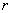, a central angle of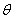radius subtends and arc whose length is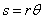.is measured in radians. Area of a sector: The areaof the sector of a circle of radiusformed by a central angleradians is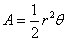where the unit of anglemust be in radians.

Rapid Study Kit for "Title":
 Flash Movie Flash Game Flash Card Core Concept Tutorial Problem Solving Drill Review Cheat Sheet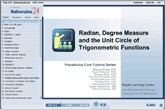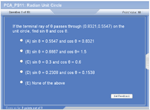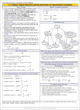"Title" Tutorial Summary : This tutorial starts with the unit circle in introducing trigonometric functions. It covers angles and the relation of angles with respect to radians and trigonometric functions. The angle measure is represented in different ways as it is shown in the examples. The properties of angles are mentioned along with their use in trigonometry problems.  Arc length and area of a sector are also discussed.

 Tutorial Features: Specific Tutorial Features: • Example problems with step by step solutions and accompanying diagrams to help illustrate the usage of angles in finding the values of trigonometric functions. Series Features: • Concept map showing inter-connections of new concepts in this tutorial and those previously introduced. • Definition slides introduce terms as they are needed. • Visual representation of concepts • Animated examples—worked out step by step • A concise summary is given at the conclusion of the tutorial.

 "Title" Topic List: The unit circle Radian and degree angle measures Positive and negative angles Complementary and supplementary angles Arc length and area of sectors

See all 24 lessons in Pre-Calculus, including concept tutorials, problem drills and cheat sheets:
Teach Yourself Pre-Calculus Visually in 24 Hours